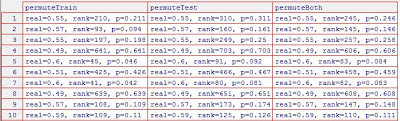## Friday, February 8, 2013

### comparing null distributions: changing the bias

Here is another example in this series of simulations exploring the null distributions resulting from various permutation tests. In this case I changed the "bias": a higher bias makes the samples easier to classify since the random numbers making up each class are drawn from a normal distribution with standard deviation 1 and mean either bias or (-1 * bias). The random seeds are the same here as in the previous examples, so the distributions are comparable.

As before, here are the null distributions resulting from ten simulations using either a bias of 0.05 or 0.15.

These are from using two runs:

and these from using four runs:

The null distributions within each pane pretty much overlap: the curves don't change as much with the different biases as they do with changing the number of runs or the permutation scheme.

The true-labeled accuracy and so the p-values change quite a lot, though:with two runs, bias = 0.05with four runs, bias = 0.05
As in the simulations with more signal (bias = 0.15), there is more variability in true-labeled accuracy in the dataset with two runs than the one with four runs. Some of the two-run simulations (#5 and #8) have accuracy below chance, and so p-values > 0.9. But two other two-run simulations (#3 and #7) have accuracy above 0.7 and p-values of better than 0.05. The best four-run simulation accuracy is 0.6, and none have p-values better than 0.05 (looking at permuteBoth).

So, in these simulations, changing the amount of difference between the classes did not substantially change the null distributions (particularly when permuting the entire dataset together). Is this sensible? I'm still thinking, but it does strike me as reasonable that if the relabeling fully disrupts the class structure then the amount of signal actually in the data should have less of an impact on the null distribution than other aspects of the data such as number of examples.